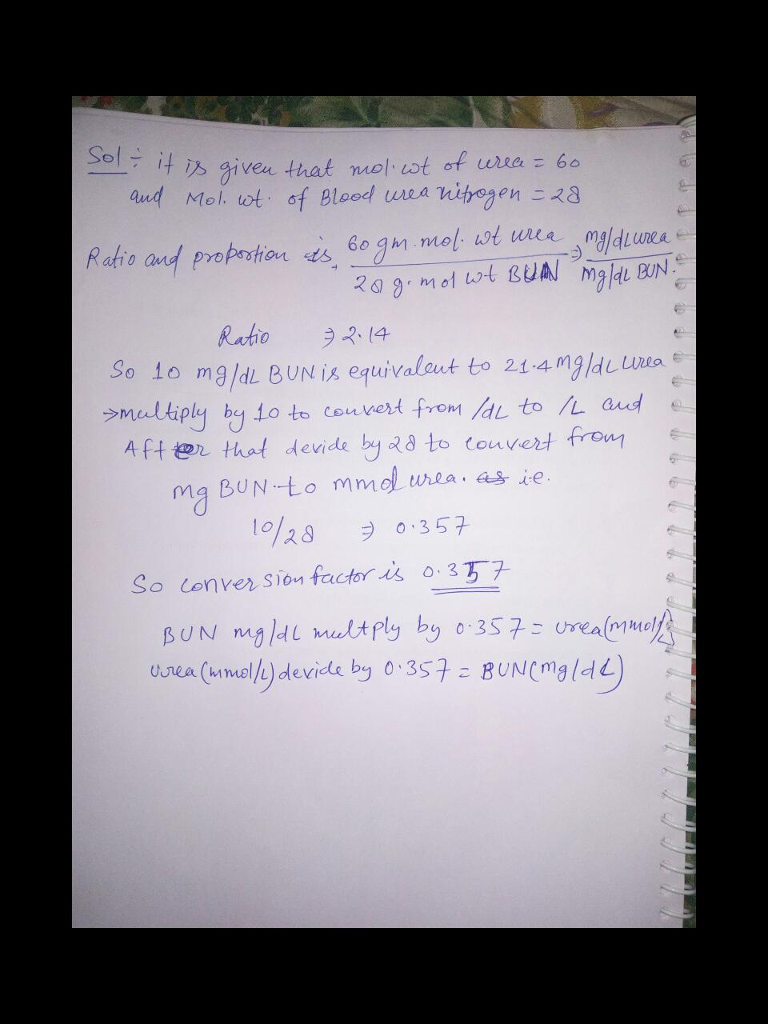# Question & Answer: Needed information for the problem Conversion problem: The molecular weight of urea…..

Needed information for the problem

Conversion problem: The molecular weight of urea [CO (NH2)2​] is 60. The molecular weight of blood urea nitrogen/serum urea nitrogen (BUN, N2) is 28.

Don't use plagiarized sources. Get Your Custom Essay on
Question & Answer: Needed information for the problem Conversion problem: The molecular weight of urea…..
GET AN ESSAY WRITTEN FOR YOU FROM AS LOW AS \$13/PAGE

Ratio and proportion problem: 60 g. mol. wt Urea/28 g. mol. wt BUN = mg/dL Urea/ mg/dL BUN

Problem

If blood urea nitrogen is measured instead of urea, what would be the conversion factor to convert a BUN to a urea in mg/dL?